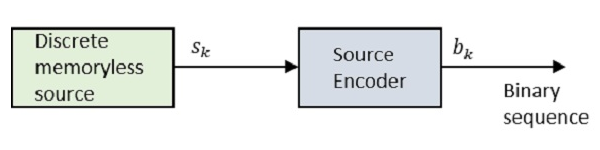# Source Coding Theorem

The Code produced by a discrete memoryless source, has to be efficiently represented, which is an important problem in communications. For this to happen, there are code words, which represent these source codes.

For example, in telegraphy, we use Morse code, in which the alphabets are denoted by Marks and Spaces. If the letter E is considered, which is mostly used, it is denoted by “.” Whereas the letter Q which is rarely used, is denoted by “--.-”

Let us take a look at the block diagram.Where Sk is the output of the discrete memoryless source and bk is the output of the source encoder which is represented by 0s and 1s.

The encoded sequence is such that it is conveniently decoded at the receiver.

Let us assume that the source has an alphabet with k different symbols and that the kth symbol Sk occurs with the probability Pk, where k = 0, 1…k-1.

Let the binary code word assigned to symbol Sk, by the encoder having length lk, measured in bits.

Hence, we define the average code word length L of the source encoder as

$$\overline{L} = \displaystyle\sum\limits_{k=0}^{k-1} p_kl_k$$

L represents the average number of bits per source symbol

If $L_{min} = \: minimum \: possible \: value \: of \: \overline{L}$

Then coding efficiency can be defined as

$$\eta = \frac{L{min}}{\overline{L}}$$

With $\overline{L}\geq L_{min}$ we will have $\eta \leq 1$

However, the source encoder is considered efficient when $\eta = 1$

For this, the value $L_{min}$ has to be determined.

Let us refer to the definition, “Given a discrete memoryless source of entropy $H(\delta)$, the average code-word length L for any source encoding is bounded as $\overline{L} \geq H(\delta)$."

In simpler words, the code word (example: Morse code for the word QUEUE is -.- ..- . ..- . ) is always greater than or equal to the source code (QUEUE in example). Which means, the symbols in the code word are greater than or equal to the alphabets in the source code.

Hence with $L_{min} = H(\delta)$, the efficiency of the source encoder in terms of Entropy $H(\delta)$ may be written as

$$\eta = \frac{H(\delta)}{\overline{L}}$$

This source coding theorem is called as noiseless coding theorem as it establishes an error-free encoding. It is also called as Shannon’s first theorem.